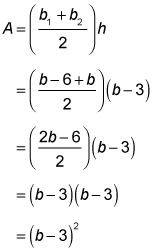##### SATEven though quadrilaterals have four sides, they can still look very different from each other. For example, the SAT Math exam may give you a question that involves anything from a square or rectangle, to a rhombus, to a parallelogram, to a trapezoid.

The following practice questions ask you to find the areas of a parallelogram and a trapezoid.

## Practice questions

1. What is the area of this parallelogram?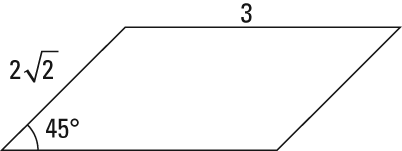A. 4 B. 5 C. 6 D. 8
2. If the trapezoid,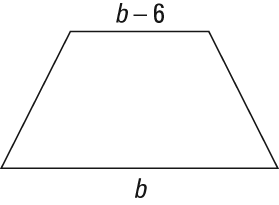has a height of b – 3, what is the area of the trapezoid?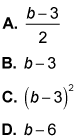1. The correct answer is Choice (C). The area of a parallelogram comes from the base times the height. The height is the perpendicular distance between the top and the bottom, not the length of the slanted side. The base is 3. To find the height, look at the 45-degree angle. This tells you that the left-hand side of the parallelogram is a 45-45-90 triangle, which has a side-length ratio of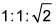where 1 is the leg and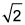is the hypotenuse.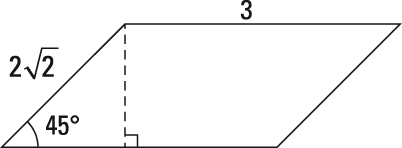If the hypotenuse of the triangle is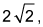each side of the triangle is 2, making the height of the parallelogram 2 and the area 6: A = bh = 3(2) = 6
2. The correct answer is Choice (C). You can find the area of a trapezoid with the formula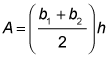where b1 represents one base, b2 represents the other base, and h represents the height. To find the area, plug in the bases and the height: